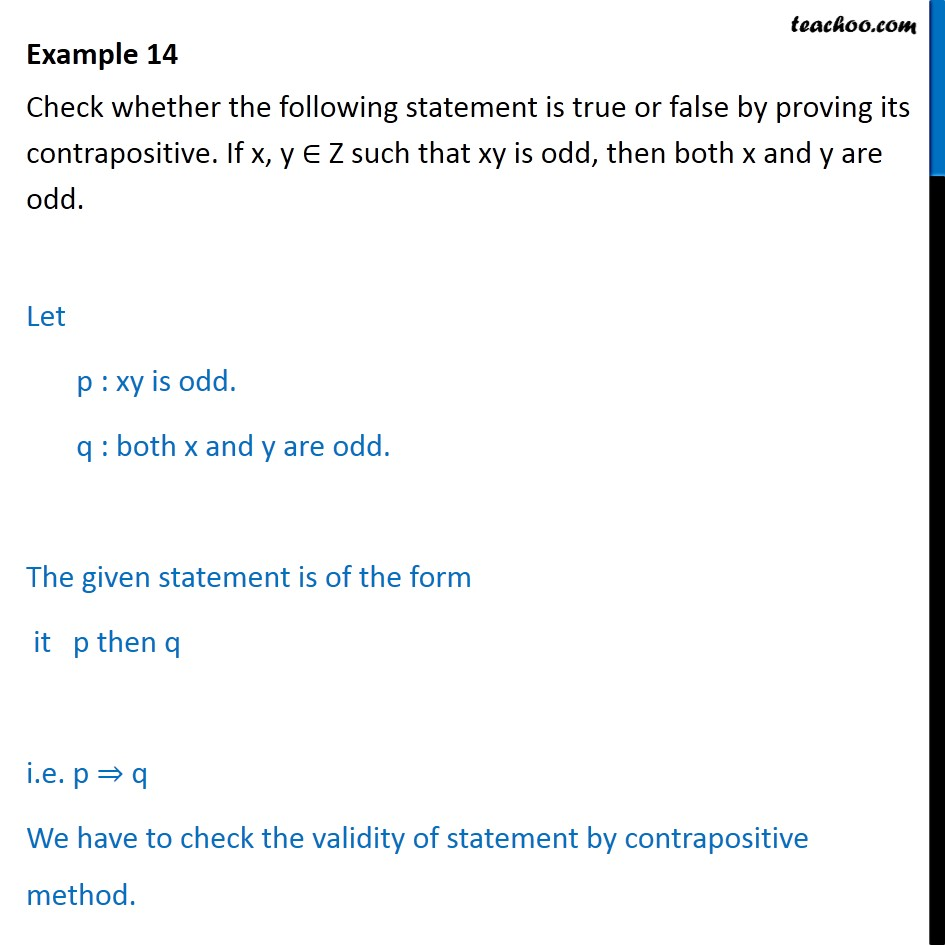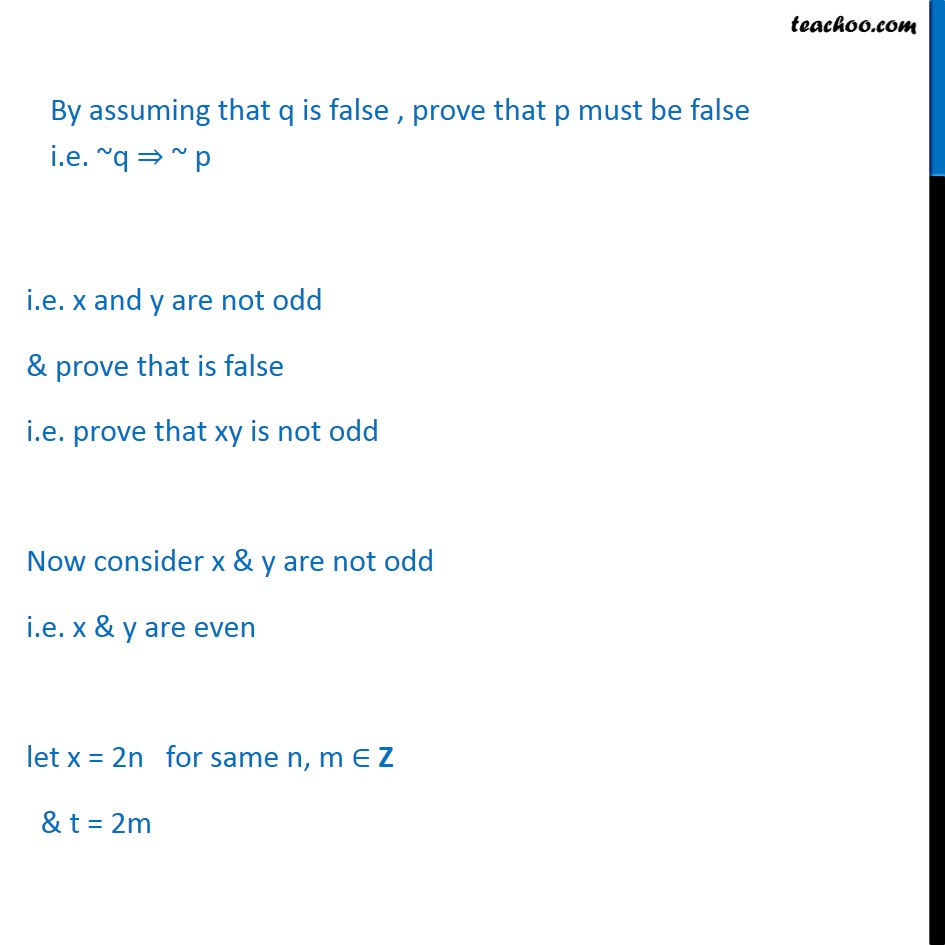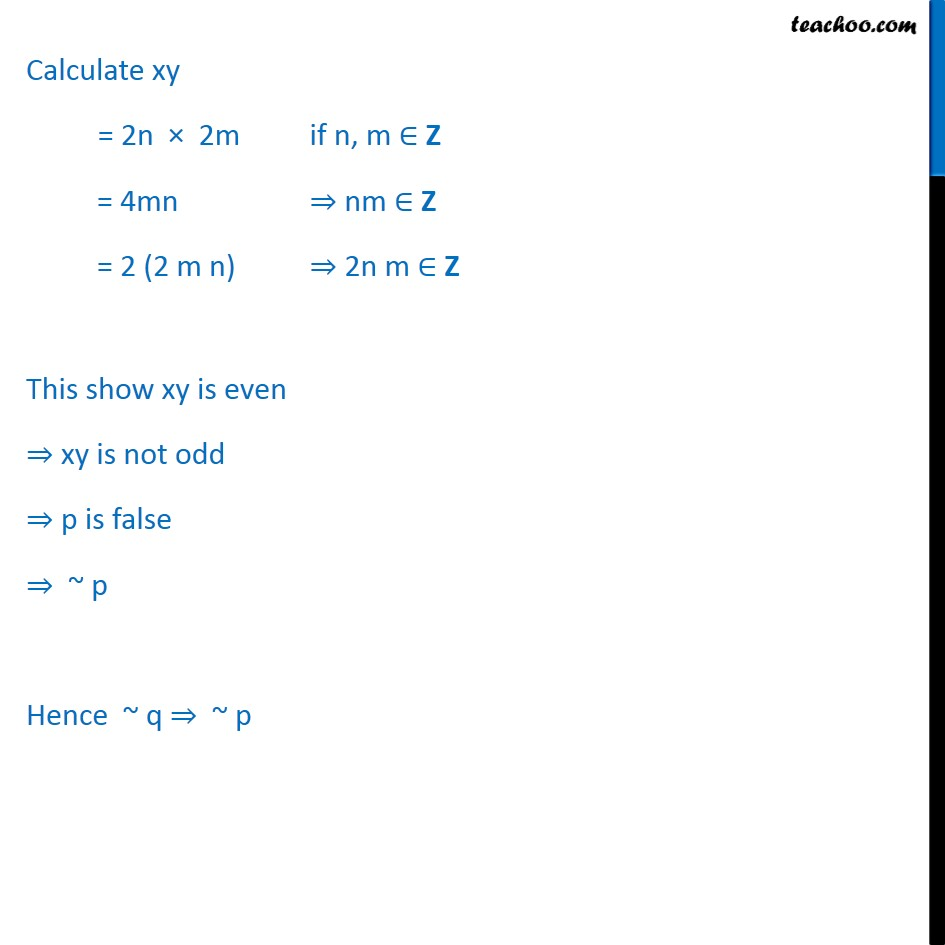1. Chapter 14 Class 11 Mathematical Reasoning (Deleted)
2. Concept wise

Transcript

Example 14 Check whether the following statement is true or false by proving its contrapositive. If x, y ∈ Ζ such that xy is odd, then both x and y are odd. Let p : xy is odd. q : both x and y are odd. The given statement is of the form it p then q i.e. p ⇒ q We have to check the validity of statement by contrapositive method. i.e. x and y are not odd & prove that is false i.e. prove that xy is not odd Now consider x & y are not odd i.e. x & y are even let x = 2n for same n, m ∈ Z & t = 2m Calculate xy = 2n × 2m if n, m ∈ Z = 4mn ⇒ nm ∈ Z = 2 (2 m n) ⇒ 2n m ∈ Z This show xy is even ⇒ xy is not odd ⇒ p is false ⇒ ~ p Hence ~ q ⇒ ~ p